# Area between two rectangles Online Quiz

Following quiz provides Multiple Choice Questions (MCQs) related to Area between two rectangles. You will have to read all the given answers and click over the correct answer. If you are not sure about the answer then you can check the answer using Show Answer button. You can use Next Quiz button to check new set of questions in the quiz.Q 1 - Find the area between the following pair of rectangles.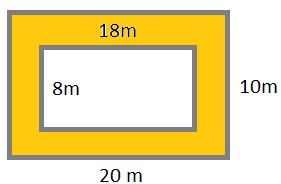### Explanation

Step 1:

Area of a rectangle = l × w; l = length; w = width

Step 2:

Area between the given pair of rectangles = 20 × 10 − 18 × 8

= 200 – 144

= 56 square m

Q 2 - Find the area between the following pair of rectangles.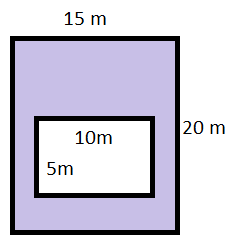### Explanation

Step 1:

Area of a rectangle = l × w; l = length; w = width

Step 2:

Area between the given pair of rectangles = 20 × 15 − 10 × 5

= 300 – 50

= 250 square m

Q 3 - Find the area between the following pair of rectangles.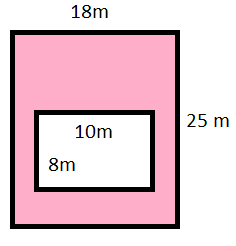### Explanation

Step 1:

Area of a rectangle = l × w; l = length; w = width

Step 2:

Area between the given pair of rectangles = 25 × 18 − 10 × 8

= 450 – 80

= 370 square m

Q 4 - Find the area between the following pair of rectangles.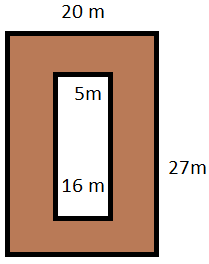### Explanation

Step 1:

Area of a rectangle = l × w; l = length; w = width

Step 2:

Area between the given pair of rectangles = 27 × 20 − 16 × 5

= 540 – 80

= 460 square m

Q 5 - Find the area between the following pair of rectangles.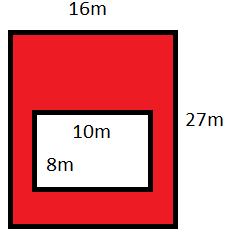### Explanation

Step 1:

Area of a rectangle = l × w; l = length; w = width

Step 2:

Area between the given pair of rectangles = 27 × 16 − 10 × 8

= 432 – 80

= 352 square m

Q 6 - Find the area between the following pair of rectangles.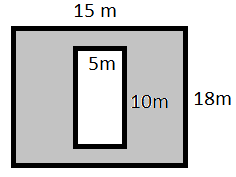### Explanation

Step 1:

Area of a rectangle = l × w; l = length; w = width

Step 2:

Area between the given pair of rectangles = 18 × 15 − 10 × 5

= 270 – 50

= 220 square m

Q 7 - Find the area between the following pair of rectangles.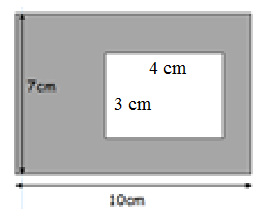### Explanation

Step 1:

Area of a rectangle = l × w; l = length; w = width

Step 2:

Area between the given pair of rectangles = 10 × 7 − 3 × 4

= 70 – 12

= 58 square cm

Q 8 - Find the area between the following pair of rectangles.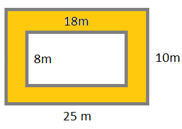### Explanation

Step 1:

Area of a rectangle = l × w; l = length; w = width

Step 2:

Area between the given pair of rectangles = 25 × 10 − 18 × 8

= 250 – 144

= 106 square m

Q 9 - Find the area between the following pair of rectangles.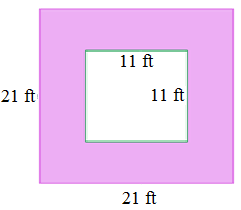### Explanation

Step 1:

Area of a rectangle = l × w; l = length; w = width

Step 2:

Area between the given pair of rectangles = 21 × 21 − 11 × 11

= 441 – 121

= 320 square ft

Q 10 - Find the area between the following pair of rectangles.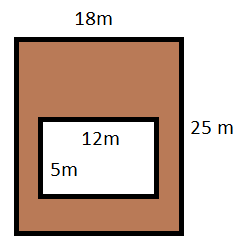### Explanation

Step 1:

Area of a rectangle = l × w; l = length; w = width

Step 2:

Area between the given pair of rectangles = 25 × 18 − 12 × 5

= 450 – 60

= 390 square m

area_between_two_rectangles.htm